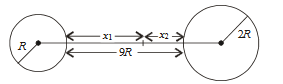#### Two spherical bodies of mass M and 5M and radii  R and 2R respectively are released in free space with initial separation between their centres equal to 12 R. If they attract each other due to gravitational force only, then the distance covered by the smaller body just before collision is Option 1)Option 2)Option 3)Option 4)As we learnt in

Let the spheres collide after time , when the smaller sphere covered distance and bigger sphere covered distance .

The gravitational force acting between two spheres depends on the distance which is a variable quantity.

The gravitational force,

Acceleration of smaller body,

Acceleration of bigger body,

From equation of motion,We know that

Therefore the two spheres collide when the smaller sphere covered the distance of

Option 1)Incorrect

Option 2)Incorrect

Option 3)Correct

Option 4)Incorrect

##### Posted by